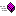XLL+ Class Library (7.0)

# matrix<T> Methods

The methods of the matrix<T> class are listed here.

#### Constructionmatrix Constructs a new matrixoperator= Assignment operator

#### Operationsadd_col Add a single column to the matrix and populate itadd_row Add a single row to the matrix and populate itclear Empty the matrixresize Resize the matrix, optionally preserving existing valuestranspose Transpose the matrix along its major diagonal

#### Attributescols Returns the number of columnsrows Returns the number of rows

#### Accessat Returns a reference to an item in the matrixcol Returns a copy of a complete columncol_ptr Returns a pointer to the data in a columnfind_in_col Searches one column for a valuefind_in_row Searches one row for a valueget_col_ptrs Populates a vector of column pointersrow Returns a copy of a complete row